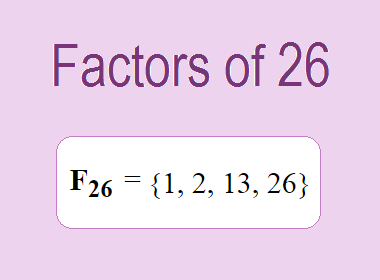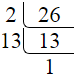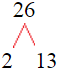# Factors of 26The factors of 26 are 1, 2, 13, and 26 i.e. F26 = {1, 2, 13, 26}. The factors of 26 are all the numbers that can divide 26 without leaving a remainder.

We can check if these numbers are factors of 26 by dividing 26 by each of them. If the result is a whole number, then the number is a factor of 26. Let's do this for each of the numbers listed above:

·        1 is a factor of 26 because 26 divided by 1 is 26.

·        2 is a factor of 26 because 26 divided by 2 is 13.

·        13 is a factor of 26 because 26 divided by 13 is 2.

·        26 is a factor of 26 because 26 divided by 26 is 1.

## How to Find Factors of 26?

1 and the number itself are the factors of every number. So, 1 and 26 are two factors of 26. To find the other factors of 26, we can start by dividing 26 by the numbers between 1 and 26. If we divide 26 by 2, we get a remainder of 0. Therefore, 2 is a factor of 26. If we divide 26 by 3, we get a remainder of 2. Therefore, 3 is not a factor of 26.

Next, we can check if 4 is a factor of 26. If we divide 26 by 4, we get a remainder of 2. Therefore, 4 is not a factor of 26. We can continue this process for all the possible factors of 26.

Through this process, we can find that the factors of 26 are 1, 2, 13, and 26. These are the only numbers that can divide 26 without leaving a remainder.

********************

********************

## Properties of the Factors of 26

The factors of 26 have some interesting properties. One of the properties is that the sum of the factors of 26 is equal to 42. We can see this by adding all the factors of 26 together:

1 + 2 + 13 + 26 = 42

Another property of the factors of 26 is that they are all prime numbers except 1 and 26.

## Applications of the Factors of 26

The factors of 26 have several applications in mathematics. One of the applications is in finding the highest common factor (HCF) of two or more numbers. The HCF is the largest factor that two or more numbers have in common. For example, to find the HCF of 26 and 39, we need to find the factors of both numbers and identify the largest factor they have in common. The factors of 26 are 1, 2, 13, and 26. The factors of 39 are 1, 3, 13, and 39. The largest factor that they have in common is 13. Therefore, the HCF of 26 and 39 is 13.

Another application of the factors of 26 is in prime factorization. Prime factorization is the process of expressing a number as the product of its prime factors. The prime factors of 26 are 2 and 13, since these are the only prime numbers that can divide 26 without leaving a remainder. Therefore, we can express 26 as:

26 = 2 × 13

We can do prime factorization by division and factor tree method also. Here is the prime factorization of 26 by division method,26 = 2 × 13

Here is the prime factorization of 26 by the factor tree method,26 = 2 × 13## Conclusion

The factors of 26 are the numbers that can divide 26 without leaving a remainder. The factors of 26 are 1, 2, 13, and 26. The factors of 26 have some interesting properties, such as having a sum of 42. The factors of 26 have several applications in mathematics, such as finding the highest common factor and prime factorization.